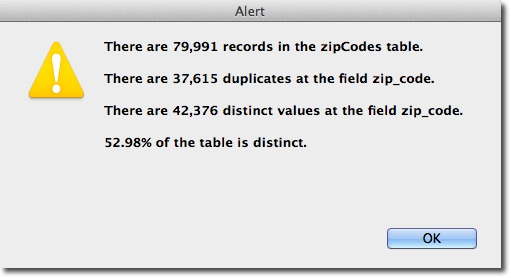KNOWLEDGE BASE
Tech Tip: A utility method to find how many duplicates are in a table using SQL DISTINCT
PRODUCT: 4D | VERSION: 12.3 | PLATFORM: Mac & Win
Published On: January 6, 2012

Ever wished for an easy way to find out how many duplicates exist in a table? Consider the dialog below:The included utility method DistinctsAndDuplicates, listed at the bottom of this Tech Tip, will produce the alert shown above with statistics shown. It is pure 4D SQL and provides one example of how to use the SQL DISTINCT clause/keyword in conjuction with the SQL COUNT function.

The DISTINCT clause allows you to remove duplicates from the result set. The DISTINCT clause can only be used with select statements. See code example below:

 ARRAY TEXT(\$zip_code_aT;0)Begin SQL   SELECT DISTINCT zip_code   FROM zipCodes   INTO :\$zip_code_aT;End SQL

Whereas some other SQL database vendors support using DISTINCT with multiple columns, 4D SQL only supports the use of DISTINCT with one column in the SELECT statement.

 If (True)   If (False)      //***************************************************      //      //   DistinctsAndDuplicates( ->\$Field )      //      //   Purpose: Document how many duplicate records are in a table      //      //   \$1 - Pointer - Pointer to a field      //      //***************************************************   End if    C_TEXT(\$MethodName_T)   \$MethodName_T:=Current method name   //===========      Declare Variables      ================   //method_parameters_declarations   C_POINTER(\$Field_P;\$1)   //----------------------------------------------------   //local_variable_declarations   C_LONGINT(\$Cnt;\$RIT;\$TableNum_L;\$FieldNum_L)   C_TEXT(\$VarName_T;\$Table_T;\$Field_T;\$SQL_T;\$Msg_T)   C_REAL(\$Pcnt_R)End if    //============      Initialize and Setup      ==============   //   Make sure the pointer points to a field   //{\$Field_P:=\$1RESOLVE POINTER(\$Field_P;\$VarName_T;\$TableNum_L;\$FieldNum_L)If ((\$TableNum_L>0) & (\$FieldNum_L>0) & (\$VarName_T=""))   //}   \$Table_T:=Table name(\$TableNum_L)   \$Field_T:=Field name(\$Field_P)   //===========      Method Actions      =====================   //   Get the percentage of distinct values   //{   \$SQL_T:="SELECT COUNT(DISTINCT("+\$Field_T+"))/COUNT(*)\r"   \$SQL_T:=\$SQL_T+"FROM "+\$Table_T+"\r"   \$SQL_T:=\$SQL_T+"INTO :\$Pcnt_R;"   Begin SQL   EXECUTE IMMEDIATE :\$SQL_T   End SQL   //}   //   Get the number of records in the table   //{   \$SQL_T:="SELECT COUNT(*)\r"   \$SQL_T:=\$SQL_T+"FROM ["+\$Table_T+"]\r"   \$SQL_T:=\$SQL_T+"INTO :\$RIT;"   Begin SQL   EXECUTE IMMEDIATE :\$SQL_T   End SQL   //}   //   Get the number of distinct field values   //{   \$SQL_T:="SELECT COUNT(DISTINCT(["+\$Field_T+"]))\r"   \$SQL_T:=\$SQL_T+"FROM ["+\$Table_T+"]\r"   \$SQL_T:=\$SQL_T+"INTO :\$Cnt;"   Begin SQL   EXECUTE IMMEDIATE :\$SQL_T   End SQL   //}   \$Msg_T:="There are "+String(\$RIT;"###,###,###,##0")+" records in the "+\$Table_T+" table.\r"   Case of       : (\$Cnt=0)         \$Msg_T:=\$Msg_T+"There are no duplicates at the "+\$Field_T+" field.\r"      : (\$Cnt=1)         \$Msg_T:=\$Msg_T+"There is one duplicate at the "+\$Field_T+" field.\r"   Else       \$Msg_T:=\$Msg_T+"There are "+String(\$Cnt;"###,###,###,##0")+" duplicates at the "+\$Field_T+" field.\r"   End case    \$Msg_T:=\$Msg_T+String(Round(\$Pcnt_R*100;2);"##0.00")+"% of the table is distinct."   ALERT(\$Msg_T)   //============      Clean up and Exit      =================Else    ALERT("Bad field pointer!")End if